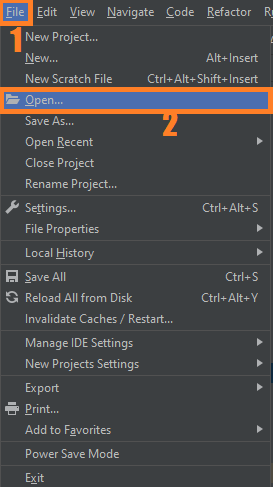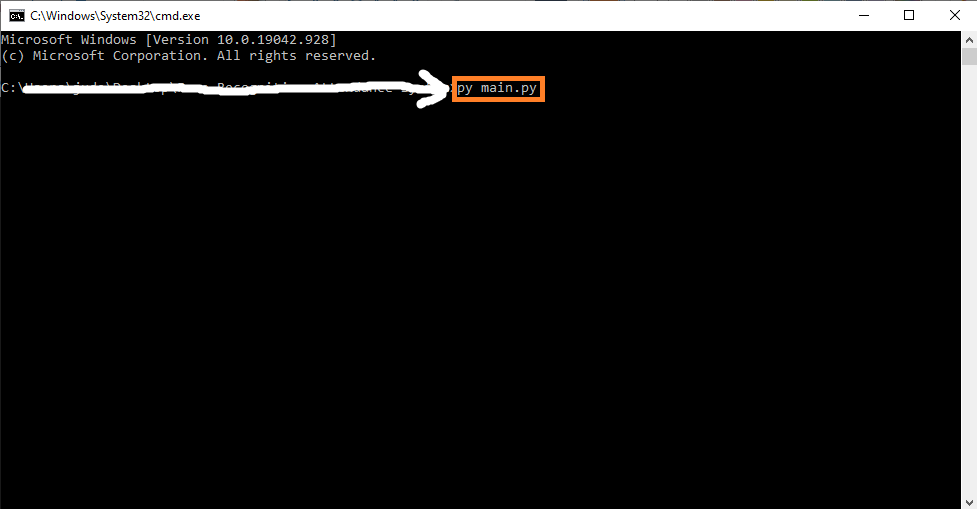# TrackBar OpenCV Python With Source Code

## TrackBar OpenCV Python With Source Code

The TrackBar OpenCV Python was developed using Python OpenCV, Trackbar is a GUI element that let the user to select a specific color value within a range of values by sliding a slider linearly. It’s similar to scrolling but it limits the user to select a specific value with its minimum and maximum limits.

## What is OpenCV?

OpenCV (Open Source Computer Vision Library) is an open-source computer vision and machine learning software library. OpenCV was built to provide a common infrastructure for computer vision applications and to accelerate the use of machine perception in commercial products.

A trackbar in OpenCV provides cv2.createTrackbar() function, to read the current position of the trackbar slider you can use cv2.getTrackbarPos() function to change the position of trackbar use cv2.setTrackbarPos().

By the way if you are new to python programming and you don’t know what would be the the Python IDE to use, I have here a list of Best Python IDE for Windows, Linux, Mac OS that will suit for you. I also have here How to Download and Install Latest Version of Python on Windows.

To start executing TrackBar OpenCV Python With Source Code, make sure that you have installed Python 3.9 and PyCharm in your computer.

## TrackBar OpenCV Python With Source Code : Steps on how to run the project

Time needed: 5 minutes.

These are the steps on how to run TrackBar OpenCV Python With Source Code

First, download the given source code below and unzip the source code.• Step 2: Import the project to your PyCharm IDE.• Step 3: Run the project.

last, run the project with the command “py main.py”## Installed Libraries

```import cv2 as cv
import numpy as np
from matplotlib import pyplot as plt```

## Complete Source Code

```import cv2 as cv
import numpy as np
from matplotlib import pyplot as plt

def change(x):
# print(x)
pass

def trackbar():
img = np.zeros((300, 512, 3), np.uint8)
cv.namedWindow("image")

cv.createTrackbar('B', 'image', 0, 255, change)
cv.createTrackbar('G', 'image', 0, 255, change)
cv.createTrackbar('R', 'image', 0, 255, change)

switch = '0: OFF\n 1: ON'
cv.createTrackbar(switch, 'image', 0, 1, change)

while True:
cv.imshow("image", img)
k = cv.waitKey(1) & 0xFF
if k == 27:
break

b = cv.getTrackbarPos('B', 'image')
g = cv.getTrackbarPos('G', 'image')
r = cv.getTrackbarPos('R', 'image')
s = cv.getTrackbarPos(switch, 'image')

if s == 0:
img[:] = 0
else:
img[:] = [b, g, r]

cv.destroyAllWindows()

if __name__ == '__main__':
trackbar()
```